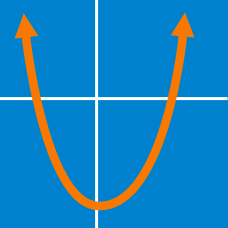Algebra

#### Challenge Quizzes

Consider the quadratic equation $7 x^2 = 49 x$. What is the sum of all the roots of this equation?

If the following statement is true, what is the value of $a$: $x^2+5x-14=(x+a)(x-2)?$

What are the roots of the quadratic equation $2(x+2)^2-33=13x+8 ?$

What are the roots of the quadratic equation $ix^2+(7-4i)x-12-12i=0 ?$

Details and assumptions

$i$ is the imaginary number that satisfies $i^2 = -1$.

If $x^2-8x+15=(x+a)(x+b),$ what is the value of $ab$?

×